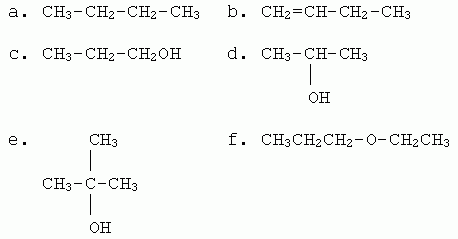## Review Quiz: Through ethers.

Ouellette 2/e through Ch 9.

 Closed book. You can use a note sheet of the type that you would use for a test, if you want. However, an alternative might be to try this quiz without notes, and then use the feedback you get from working on this as a guide to making your test note sheet. Level. Most of this quiz is "basic" or "core" level. Consider the following six compounds, which sample most of the types of chemicals we have covered up through Ch 9. (The main omission here is aromatic compounds.) Most of the questions below deal with this set of six chemicals. For questions that ask "which" of these has a particular feature or property, "which" may mean any number from none to all.1. Name each chemical. (I will include on the answer key both IUPAC and good common names. Any one name is acceptable.)

2. How would you classify each of these chemicals, based on their key functional groups? What is that key functional group? When relevant, include the "degree".

3. a. Which of these will burn, in the usual sense?
b. Write a balanced equation for the combustion of chemical d. Include phases.
c. Which other chemical, above, would have the same balanced equation for its combustion as in part b? Why?

4. For this question, ignore the alcohols in the set of chemicals. For each of the others, what type of reaction is typical of this type of chemical?

5. a. Consider the common reaction of reacting with H2 in the presence of a noble metal catalyst. Which compound(s) will react? Give the product.
b. Which of the following terms, used to describe reaction types, would apply in this case: addition, hydrogenation, reduction?

6. Consider the common reaction of acid-catalyzed hydration. Which compound(s) will react? Give the major product, and explain why it is the major product.

7. Show the result of mild oxidation of the primary alcohol. Show only the first step.

8. a. Show how you could make a ketone in one step from one of the given compounds.
b. As a more advanced question... Show how you could make a ketone in two steps from another one of the given compounds.

9. Consider the alcohol that is not easily oxidized. Show the product of acid-catalyzed dehydration (elimination) of that alcohol.

10. a. Which of these compounds are acidic or basic, in the common context of affecting the pH of water?
b. Two types of compounds that we have discussed, but which are not represented above, are typically weakly acidic. What are they?

11. Consider the following general classes of compounds: hydrocarbons, ethers, alcohols. (In parts a and b, assume "all else equal". Do not consider the specific compounds listed at the top of this page.)
a. Rank them by boiling point (highest first). Explain the main reasons for the rankings.
b. Rank them by solubility in water (highest first). What is the key reason for the major difference from the rankings in part a?
c. Give an example of a specific alcohol and a specific hydrocarbon that would violate the general trend from part a. Explain why.
d. Would the compounds you suggest in part c also violate the solubility trend in part b? Explain.

 12. Consider the Figure at the right. At first glance, the compound shown there may look rather complex. However, it is very repetitive. a. Consider all the O atoms. What functional group do they represent? b. If you were going to carry out a chemical reaction on this compound, what functional group would probably be the target of the reaction? What would be the reaction type?Last update: April 14, 2019